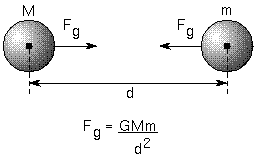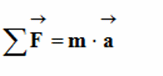Satellite is different in terms of its functions, there are satellites for communication, photography and other for weather... When we look at the satellite, a quick technical look consists of two basic parts, the first part being the function of the satellite which varies according to the tasks assigned to it and the second part cares about the satellite itself in terms of location, energy, direction, speed, and many other things as payment systems to correct the path. and others. If you want to send a space satellite you need to have a contract with a space company specializing in this area such as Space X, where you can send your satellite to the desired orbit and give it the necessary payment for its stay in orbit and also the maintenance work if it is damaged or replaced in case of loss in space. On the other hand, someone might wonder what the role of satellites is specifically in our lives? First, look at the TV, especially the TV, the remodeling and the cable, which takes advantage of the satellite to capture a wave that suddenly turns you into a picture. To look again at weather conditions, the satellite of weather surveillance captures the image and then a computer in the center Manufactures The odds of a closer end to the next weather, if you're using the navigation system directly or indirectly, you are here dealing with the satellites industrial, the non-collision of airplanes goes back to this system, the fact that many examples around us are working using satellites where as I said before I became An essential element in helping human life to live better.

### How does the satellite work in theory

Before we start anything by equations or physics we will talk about the story of the cannonWhen a cannon throws a shell, it will go as shown in the picture because of factors such as friction and ground gravity that are affected by the Earth's drift into the ground and fall. But what if there is no gravity and friction? The simple answer is that the projectile will not fall to the ground, but will go straight in a straight line which is called self-made in physics, and the projectile will tend to walk in a straight line and at regular speeds. The projectile will emerge from the ground and go infinite until it crashes into a space object.

Let's imagine a third time that this projectile is attracted by power to the Earth and is the force of gravity while another force pushes it outward, where the two forces are equal with the addition of no friction, here the shell will take orbit around the Earth and it will go an infinite way through first payment or initial velocity with the alarm that we assume that the Fully equal attraction above the whole planet. The following figure will show you how the projectile will go:

### How the satellite works physically

In this part, we're going to study the satellite's physical movement, so you'll find here a set of theories and laws that have been simplified to be understood by anyone. The powers mentioned here are important for the study of the satellite movement, so you will find in the final conclusion the work we have done.. Let's get started.

In the satellite movement there are many influential forces on it and these powers are as follows:

-Gravity of the Earth.
-Centrifugal force.
-Force of friction
-Momentum

### 1 - gravity

This is what we mean by the tendency of objects to move towards Earth if they enter in the field of gravity, and gravity in general we mean the tendency of objects to go to each other the law of Public attraction was drafted by the Newton World, where it linked the mass and the general constant attraction of KIPLPL and the distance between the two bodies which is as follows :F: Force of Gravity

G: Constant public attraction is equal to the rating of international standard system

M: Earth mass

m: The body mass that we want to measure gravitational force has the example of the satellite

d: Distance between the two bodies (between their positions)

This law must be well known to be used later in linking the rings between centrifugal force and ground gravity.

### 2 - centrifugal force

Centrifugal force is an unreal force, but we can do it as a result of the fact that objects tend to walk in a straight line and in the case of a body orbiting a circular path it is in every time he tends to get out of his way but the central attraction force (in the case of a satellite is anomalies to warn him that he's not going out. To push out the power that it causes it is a product of the Inertia of the Jedi (walking in a straight line at a constant speed called self-inertia).

Maybe the following picture shows you a good deal:

We note here that there are three things affecting the centrifugal force: the radius of the circulation, the speed of the body and its mass, which are only three variables that are in the construction of the centrifugal force equation.

F: Centrifugal force

m: Body mass

v: Body Speed

### 3. Force of friction and friction in space

The force of friction is an opposing force of the motor force and reduces the speed of the objects, which are the result of contact between two bodies, on the ground, the air acts to hinder the movement aircraft through friction with the fuselage and at the same time is an important element in its flight because without air friction the plane cannot fly. I have to make a remark here that the driving force is a force that makes the thing move may be a preliminary payment, engine or jet motive.

In space there is emptiness, what we mean by emptiness is the absence of gases, but in order to speak accurately there are very few gases where the ratio is approaching zero but generally and because we love rounding we say that there are no gases in space because of course it is its effect in the long run, so we go from this and say that it is There is friction in space and this is very important because friction as I said earlier prevents the speed and obstruction of objects which makes us need a driving force in every time to push objects but in space there's no such thing, if you push the body, it won't stop spinning, which is what I talked about in the gun story. where when We tossed the projectile, and without friction, it didn't stop completing its trajectory. (We call gases in the air in general form) so we say that the air on earth is what causes friction with flying objects and all that air holds, we're either going to take advantage of it. An example of an aircraft taking off positively or negatively affecting us in The case that we want to throw something into space and don't want it to stop so in space there is no air that is why we are saying that there is no friction.

### 4- Newton's Second Law

First of all, I'm going to say I'm not going to use Newton's law in the full form, but I'll use it to make one thing simple, but to know, Newton's second law states that "if a force or group of power affects an object, it gains an acceleration proportional to the outcome of the VIES and inversely with its mass" and writes:The benefit of this law in our study is to find out that the satellite when we leave it at an elementary speed will not stop spinning, which is our goal. is to see only the forces influencing the satellite are the only driving force which is very important in our study. Let us look at the outcome of the forces affected by an artificial satellite in its circulation.

Consider first:

F: The driving force of the satellite

B: The inhibiting force of the satellite or the force of friction

A: Centrifugal Force

P: Gravitational force Ground

We are now writing the results of the forces influencing the satellite through Newton's second law:

In order for the moon to run from an elementary speed and without stopping we need to keep only the driving force, and this is through that friction must be non-existent.

### 5- Momentum and gravity force

On the previous axis, I ended up having to make the centripetal force and gravitational force, and so to walk the moon. The industry is infinite without a motor only through elementary speed and I specifically said that we should look for a condition that we control to achieve the idea. So what I mean equal is this congruent:

And if we simplify it because as we observe the R = r and our m2 in both sides we find:

Through this equation we can figure out the speed at which the satellite should be paid to walk without a motive afterwards:

So just by knowing the constant public attraction and the mass of the earth and the distance between the center of the Earth and the satellite center you will find the speed at which the satellite must be pushed to operate without motors. A new remark is that the average density of the earth is used in the equation as an alternative to the Earth's mass and will reach a two-element equation, the radius of the earth and some known constants. So the equations may differ somewhat but the same idea is that we can run a satellite without engines but does that mean we're abandoning Full n engines?

That's not true, because sometimes in areas where the Earth's gravity changes, the satellite's attraction has affected the sun, so we have to run the impulses to correct path, the previous equation is a theoretical equation, but it provides us with a distinctive picture of the possibility of mathematical equations in building new innovations as I have to I remember that low or low-orbit satellites need to be corrected always because of a little friction factor with the atmosphere and also the warning to something else, sometimes the antennas on the satellite are only working if they're heading towards the Earth's control center, so on the satellite that He corrects his situation by paying and you wonder where the energy will come from and I'll tell you that solar energy is one of the solutions to do this.